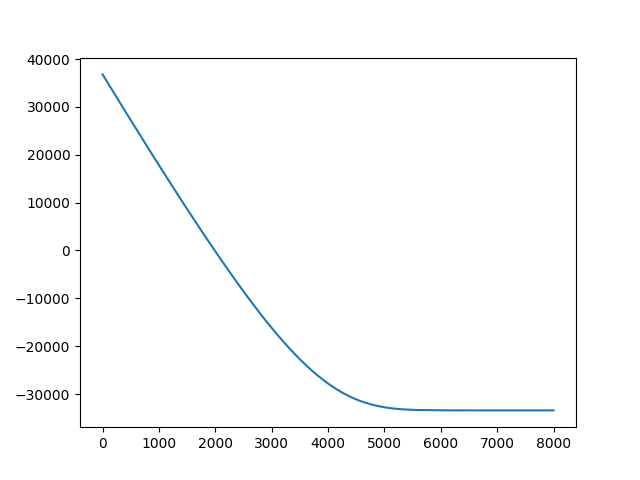# Pyro.contrib.gp

Hi, I’m using the pyro.contrib.gp to train my data, but the trace of ELBO seems irregular like the above picture shows, it dropped to -30,000.
The input and output data are all in [-1, 1], I have tried different values of variance, lengthscale and learning rate, but the situation has not improved, and the predict value of Xnew is also very poor, so I came here for help.Below is my code:

X = data_1[‘params’]
Y = data_2[‘Cp_M_neg’]
X = torch.tensor(X, dtype=torch.float)
Y = torch.tensor(Y, dtype=torch.float)
kernel = gp.kernels.RBF(input_dim=1, variance=torch.tensor(1.), lengthscale=torch.tensor(1.))
gpr = gp.models.GPRegression(X, Y.t(), kernel, noise=torch.tensor(10.))
gpr.kernel.lengthscale = pyro.nn.PyroSample(dist.LogNormal(0.0, 1.0))
gpr.kernel.variance = pyro.nn.PyroSample(dist.LogNormal(0.0, 1.0))
I’m not sure what’s wrong. Your training process looks reasonable to me. I would suggest to perform maximum likelihood (i.e. no `PyroSample`) before performing MAP estimate. You can also try some tips and tricks here.